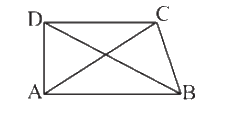# Ex.6.4 Q4 The-Triangle-and-its-Properties Solutions NCERT Maths Class 7

Go back to  'Ex.6.4'

## Question

$$ABCD$$ is a quadrilateral. Is $$AB\! +\! BC\! +\! CD \!+\! DA\! >\! AC\! +\! BD?$$Video Solution
Triangle & Its Properties
Ex 6.4 | Question 4

## Text Solution

What is known?

$$ABCD$$ is a quadrilateral. $$DB$$ and $$AC$$ are diagonals.

What is unknown?

Is $$AB \!+\! BC\!+\!CD\!+\!DA \!>\! AC\!+\!BD?$$

Reasoning:

In this question, it is asked to check Is $$AB \!+\! BC \!+\! CD \!+\! DA \!>\! AC \!+\! BD$$or not. This question is based on the property that the sum of lengths of two sides of a triangle is always greater than the third side. Now visually identify that the quadrilateral $$ABCD$$ is divided by diagonals $$AC$$ and $$BD$$ into four triangles. Now, take each triangle separately and apply the above property and then add $$\rm{}L.H.S$$ and $$\rm{}R.H.S$$ of the equation formed.

Steps:

In triangle $$ABC,$$

$$AB + BC > AC{\rm{ }} \qquad \ldots \ldots .{\rm{ }}\left( 1 \right)$$

In triangle $$ADC,$$

$$AD + CD > AC{\rm{ }} \qquad \ldots \ldots .{\rm{ }}\left( 2 \right)$$

In triangle $$ADB,$$

$$AD + AB > DB{\rm{ }} \qquad \ldots \ldots .{\rm{ }}\left( 3 \right)$$

In triangle $$DCB,$$

$$DC + CB > DB{\rm{ }} \qquad \ldots \ldots .{\rm{ }}\left( 4 \right)$$

Adding equation $$\rm{}(1),$$ $$\rm{}(2),$$ $$\rm{}(3)$$ and $$\rm{}(4)$$ we get,

\begin{align}& \left[ \begin{array}& AB\!+\!BC\!+\!\\AD\!+\!CD\!+\!\\AD\!+\!AB\!+\! \\ DC\!+\!CB \\ \end{array} \right]\!>\!\left[ \begin{array}& AC\!+\!AC+\! \\ DB\!+\!DB \\ \end{array} \right] \\ & \left[ \begin{array} & AB\!+\!AB\!+\!BC\!+\! \\ BC\!+\!CD\!+\!CD\!+\! \\ AD\!+\!AD \\ \end{array} \right]\!>\!2AC\!+\!2DB \\ & \left[ \begin{array} & 2AB\!+\!2BC\!+\! \\ 2CD\!+\!2AD \\ \end{array} \right]\!>\!2AD\!+\!2DB \\ & AB\!+\!BC\!+\!CD\!+\!AD\!>\!AC\!+\!DB \\ \end{align}

Useful Tip:

Whenever you encounter problems of this kind, it is best to think of the property based on sum of lengths of any two sides of a triangle is always greater than the third side.

Learn from the best math teachers and top your exams

• Live one on one classroom and doubt clearing
• Practice worksheets in and after class for conceptual clarity
• Personalized curriculum to keep up with school